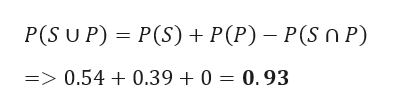# In jumbled box of pens and pencils, the probability of randomly choosing a sharpened pencil is .54 and the probability of randomly choosing a pen is .39.  Find the probability of choosing an in sharpened pencil or pen from the box.

Question
51 views

In jumbled box of pens and pencils, the probability of randomly choosing a sharpened pencil is .54 and the probability of randomly choosing a pen is .39.  Find the probability of choosing an in sharpened pencil or pen from the box.

check_circle

Step 1

Given data

probability of randomly choosing a sharpened pencil P(S) = 0.54

probability of randomly choosing a pen P(P) ...help_outlineImage TranscriptioncloseP(S U P) P(S) P(P) - P(S n P) =>0.54 0.39 0 = 0.93 fullscreen

### Want to see the full answer?

See Solution

#### Want to see this answer and more?

Solutions are written by subject experts who are available 24/7. Questions are typically answered within 1 hour.*

See Solution
*Response times may vary by subject and question.
Tagged in

### Other